# Lewis Structures Lewis Structures Lewis structures are representations

• Slides: 21Lewis Structures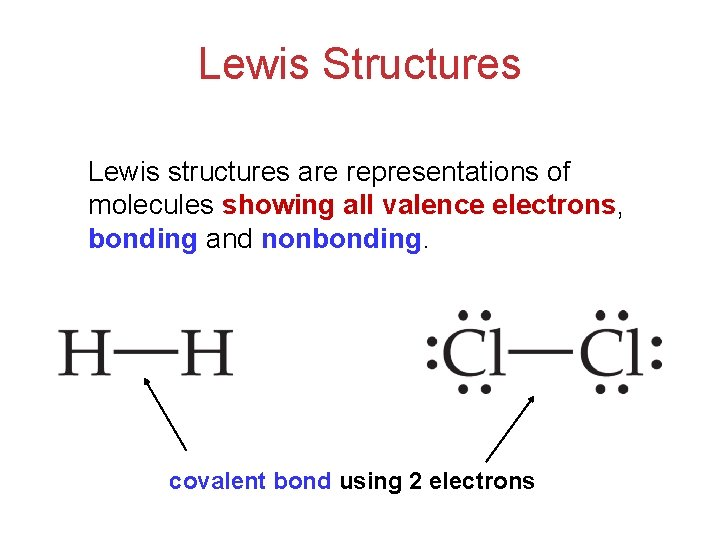Lewis Structures Lewis structures are representations of molecules showing all valence electrons, bonding and nonbonding. covalent bond using 2 electrons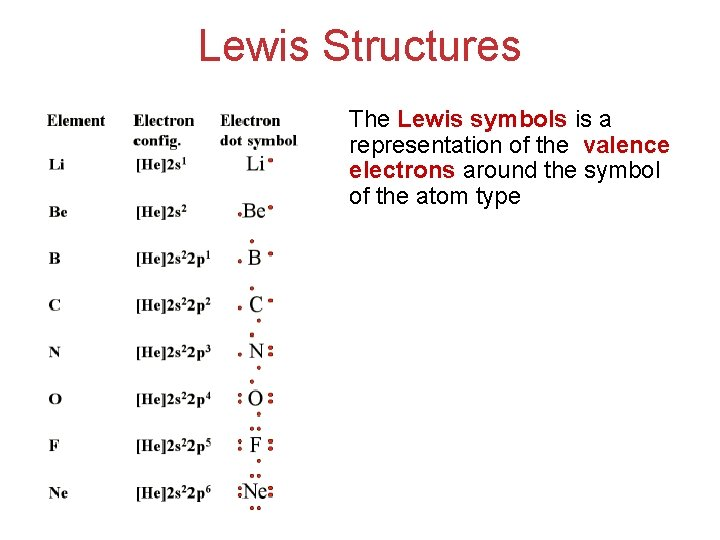Lewis Structures The Lewis symbols is a representation of the valence electrons around the symbol of the atom type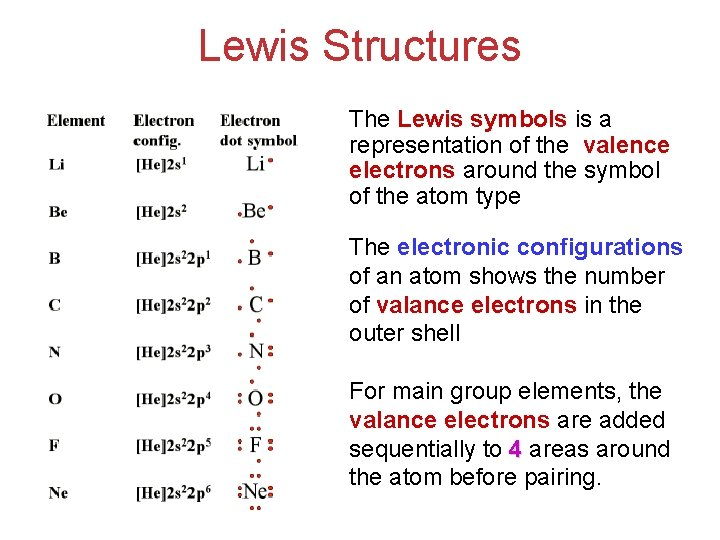Lewis Structures The Lewis symbols is a representation of the valence electrons around the symbol of the atom type The electronic configurations of an atom shows the number of valance electrons in the outer shell For main group elements, the valance electrons are added sequentially to 4 areas around the atom before pairing.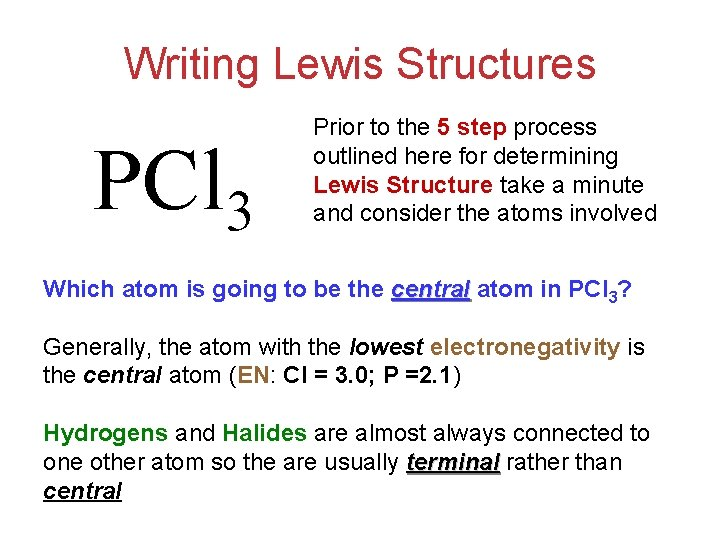Writing Lewis Structures PCl 3 Prior to the 5 step process outlined here for determining Lewis Structure take a minute and consider the atoms involved Which atom is going to be the central atom in PCl 3? Generally, the atom with the lowest electronegativity is the central atom (EN: Cl = 3. 0; P =2. 1) Hydrogens and Halides are almost always connected to one other atom so the are usually terminal rather than central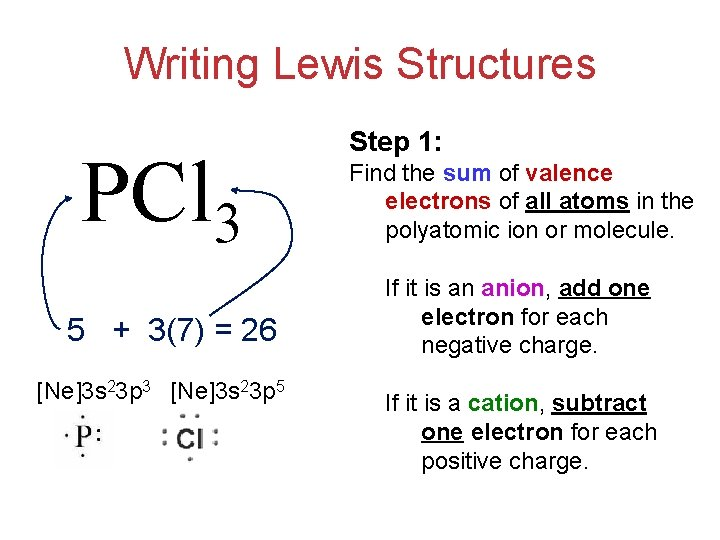Writing Lewis Structures PCl 3 5 + 3(7) = 26 [Ne]3 s 23 p 3 [Ne]3 s 23 p 5 Step 1: Find the sum of valence electrons of all atoms in the polyatomic ion or molecule. If it is an anion, add one electron for each negative charge. If it is a cation, subtract one electron for each positive charge.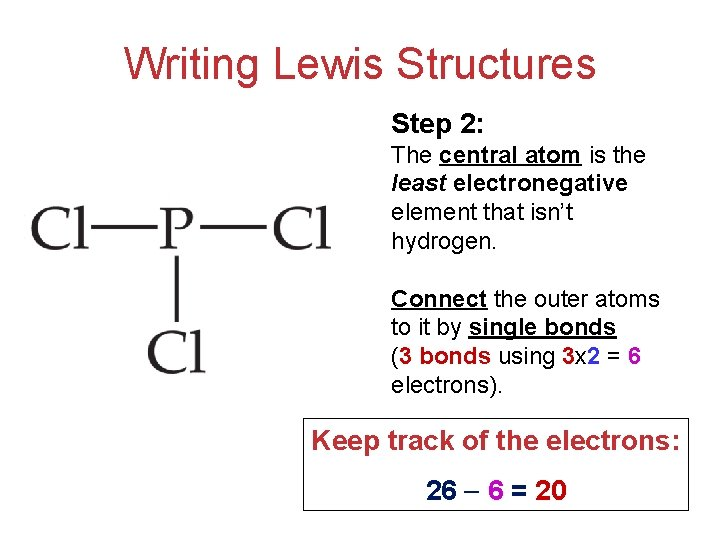Writing Lewis Structures Step 2: The central atom is the least electronegative element that isn’t hydrogen. Connect the outer atoms to it by single bonds (3 bonds using 3 x 2 = 6 electrons). Keep track of the electrons: 26 6 = 20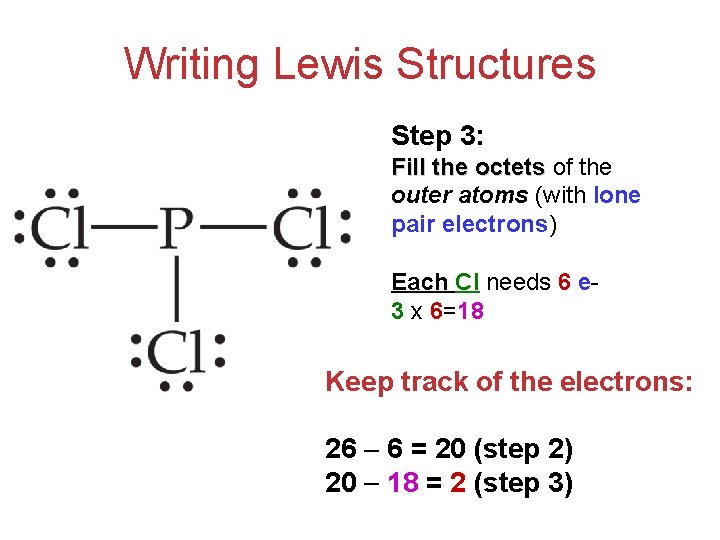Writing Lewis Structures Step 3: Fill the octets of the outer atoms (with lone pair electrons) Each Cl needs 6 e 3 x 6=18 Keep track of the electrons: 26 6 = 20 (step 2) 20 18 = 2 (step 3)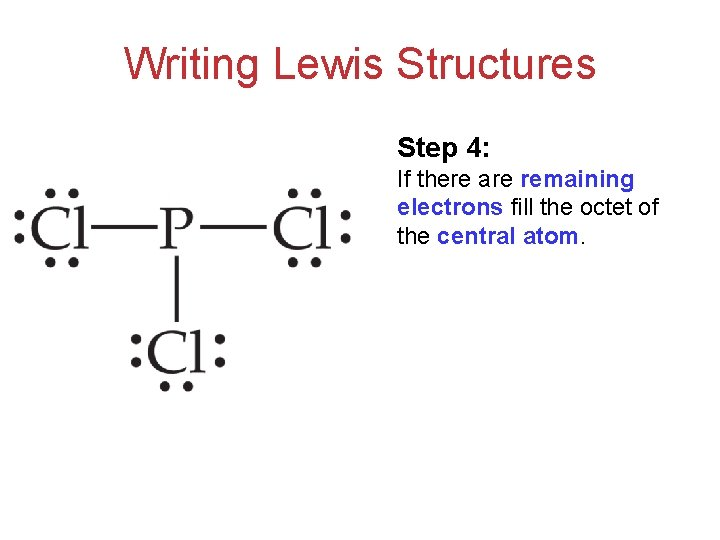Writing Lewis Structures Step 4: If there are remaining electrons fill the octet of the central atom.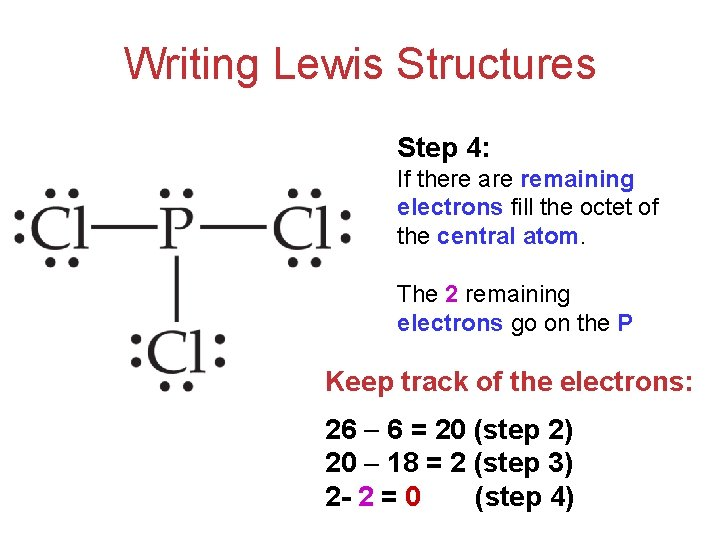Writing Lewis Structures Step 4: If there are remaining electrons fill the octet of the central atom. The 2 remaining electrons go on the P Keep track of the electrons: 26 6 = 20 (step 2) 20 18 = 2 (step 3) 2 - 2 = 0 (step 4)Writing Lewis Structures (w/ Step 5) HCN 1 +4+5 = 10 H: 1 s 1 C: [He]2 s 22 p 2 N: [He]2 s 22 p 3 Step 1: Find the sum of valence electrons of all atoms in the polyatomic ion or molecule. If it is an anion, add one electron for each negative charge. If it is a cation, subtract one electron for each positive charge.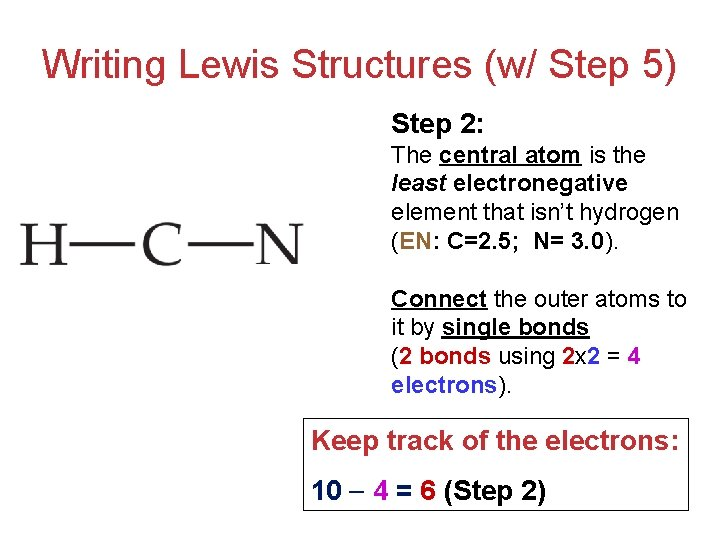Writing Lewis Structures (w/ Step 5) Step 2: The central atom is the least electronegative element that isn’t hydrogen (EN: C=2. 5; N= 3. 0). Connect the outer atoms to it by single bonds (2 bonds using 2 x 2 = 4 electrons). Keep track of the electrons: 10 4 = 6 (Step 2)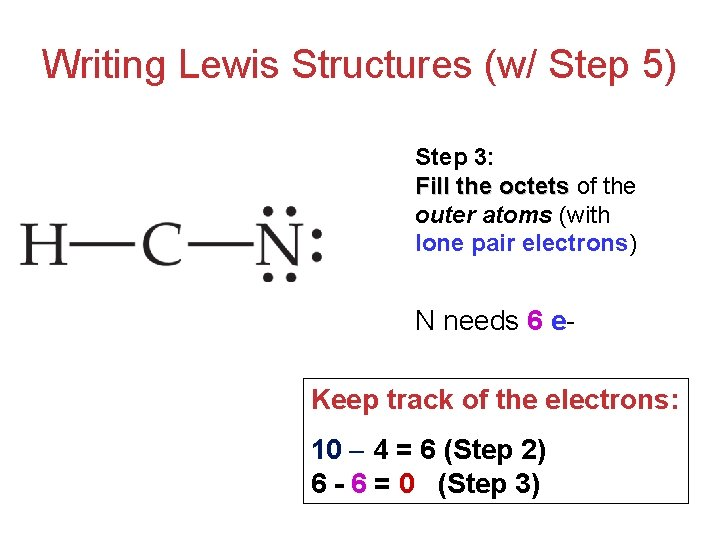Writing Lewis Structures (w/ Step 5) Step 3: Fill the octets of the outer atoms (with lone pair electrons) N needs 6 e. Keep track of the electrons: 10 4 = 6 (Step 2) 6 - 6 = 0 (Step 3)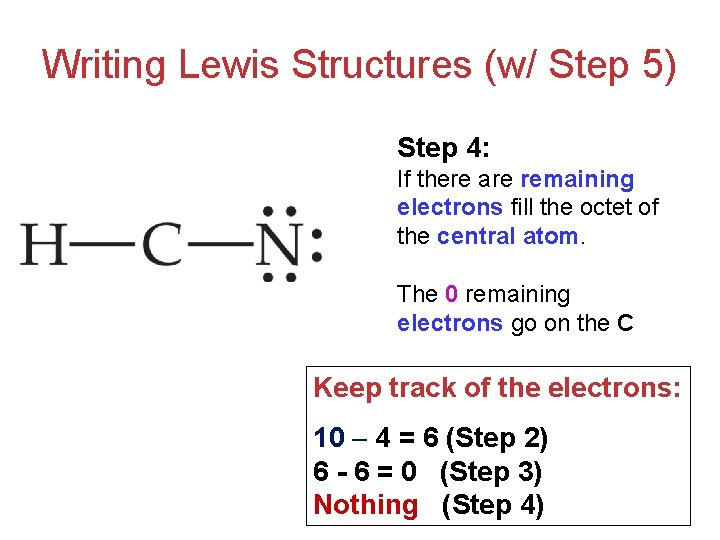Writing Lewis Structures (w/ Step 5) Step 4: If there are remaining electrons fill the octet of the central atom. The 0 remaining electrons go on the C Keep track of the electrons: 10 4 = 6 (Step 2) 6 - 6 = 0 (Step 3) Nothing (Step 4)Writing Lewis Structures (w/ Step 5) Step 5: If you run out of electrons before the central centra atom has an octet… (C only has 4 e- around it) form multiple bonds until it does by using lone pairs from terminal atomsWriting Lewis Structures • The best Lewis structure… …is the one with the fewest charges. …puts a negative charge on the most electronegative atom. CNO-2 +1 -1 -1Resonance • One Lewis structure cannot accurately depict a molecule such as ozone. • We use multiple structures, resonance structures, to describe the molecule.Exceptions to the Octet Rule Ions or molecules with less than an octet. Ions or molecules with more than eight valence electrons (an expanded octet).Fewer Than Eight Electrons If filling the octet of the central atom results in a negative charge on the central atom and a positive charge on the more electronegative outer atom, don’t fill the octet of the central atom.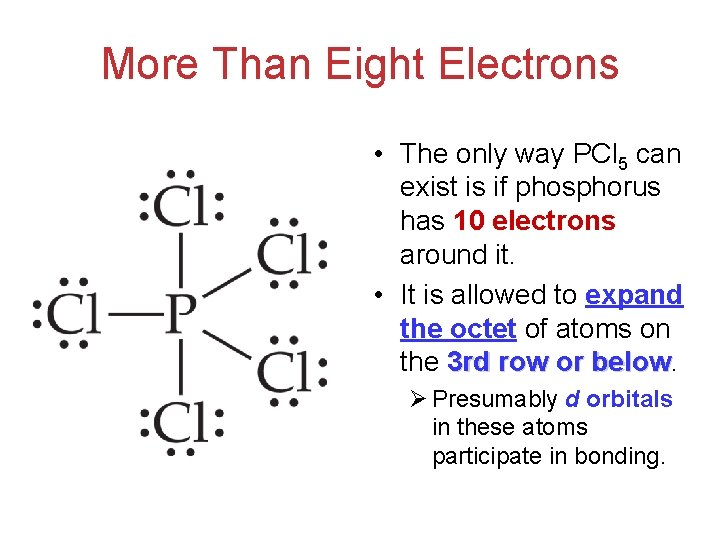More Than Eight Electrons • The only way PCl 5 can exist is if phosphorus has 10 electrons around it. • It is allowed to expand the octet of atoms on the 3 rd row or below Ø Presumably d orbitals in these atoms participate in bonding.More Than Eight Electrons We can draw a Lewis structure for the phosphate ion (PO 4)-3 [32 valence e-] that has only 8 electrons around the central phosphorus. The better structure is to put a double bond between the phosphorus and one of the oxygens to eliminates some formal charges (5 atoms w/ charges Vs. 3 atoms ).